中文版 ENGLISH
 Home About SKLFS News and Events Research People Facilities Exchange and Cooperation Related Links Sitemap
 Chen HX, Liu NA (2009b) Journal of Thermal Analysis and Calorimetry 96(1), 175-178. Date: 2011-08-16   Author: SKLFS  ,   Source: WOS  ,
 Chen HX, Liu NA (2009b) NEW APPROXIMATE FORMULAE FOR THE GENERALIZED TEMPERATURE INTEGRAL. Journal of Thermal Analysis and Calorimetry 96(1), 175-178. [In English] Keywords:approximation, kinetic analysis, non-isothermal, temperature integral, kinetics, dependenceAbstract: State Key Laboratory of Fire Science, University of Science and Technology of China, Hefei, Anhui 230026, P. R. China The generalized temperature integral integral(T)(0)T(m)exp(-E / RT)dT frequently occurs in non-isothermal kinetic analysis. Here E is the activation energy, R the universal gas constant and T the absolute temperature. The exponent m arises from the temperature dependence of the pre-exponential factor. This paper has proposed two new approximate formulae for the generalized temperature integral, which are in the following forms: h(m)(x)=x/(1.00141 + 0.00060m)x + (1.89376 + 0.95276m) h(m)(x)=x + (0.74981 - 0.06396m)/(1.00017 + 0.00013m)x + (2.73166 + 0.92246m) where h(m)(x) is the equivalent form of the generalized temperature integral. For commonly used values of m in kinetic analysis, the deviations of the new approximations from the numerical values of the integral are within 0.2 and 0.03%, respectively. In contrast to other approximations, both the present approaches are simple, accurate and can be used easily in kinetic analysis.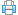Print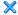Close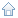State Key Laboratory of Fire Science, University of Science and Technology of China Jinzhai Road 96, Hefei, Anhui, P. R. China P. O.: 230026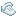Tel:(+86)551 63601651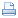Fax:(+86)551 63601669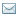E-mail:sklfs@ustc.edu.cn